Finding Maxima and Minima
(Extrema)

When studying relative (local) maxima and minima of graphs, the graphing calculator may be used to find these values.  Several methods may be used.

 Method 1: (using the CALCULATE Menu)

Locate the relative maximum/minimum for the function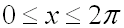.

Step 1:Enter the equation into Y=

Step 2: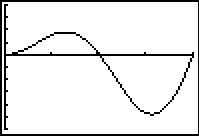Adjust the WINDOW to coincide with the given domain.  Hit GRAPH.  Be sure the graph is viewable in the graphing window.  Adjust accordingly.

Step 3: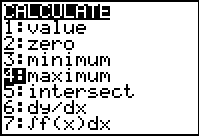Let's find the maximum value first.  Under the CALC (2nd TRACE) menu, choose #4 maximum.
Hit ENTER

Step 4: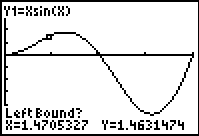When asked for Left Bound, move the cursor (use arrow keys) to the left of the observed maximum location.  Hit ENTER.  You will see a mark indicating that you have "locked" this position.

Step 5:When asked for Right Bound, move the cursor (use arrow keys) to the right of the observed maximum location.  Hit ENTER.  You will see a mark indicating that you have "locked" this position.

Step 6:When asked for Guess, simply hit ENTER.

Step 7: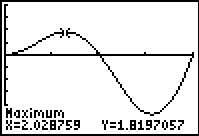The coordinates of the maximumm value (within your marked boundaries) will appear.
Max (2.029, 1.920)

 Note:   If you are investigating more than one location on a          graph, you must start this process from the beginning for each point being investigated. (Return to Step 3.)

This same process is used to examine relative minima.
(#3: minimum)

 Method 2: (using the fMin and fMax functions)

Investigate relative minima/maxima for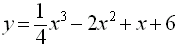.

Step 1: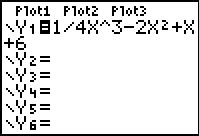Enter the equation into Y=

Step 2: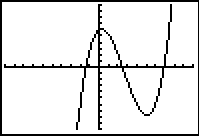Hit GRAPH.  Be sure the graph is viewable in the graphing window.  Adjust the WINDOW if needed.

Step 3: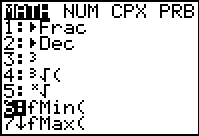From the HOME screen, hit the MATH key.  Choose either #6 fMin or #7 fMax.
Hit ENTER

Step 4: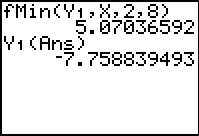The parameters for fMin and fMax the same:fMin(expression, variable, left bound, right bound)
Be careful:
The answer from fMin is the X-coordinate where the minimum occurs.  It is not the actual y-value minimum.   You must then calculate the y-value.

Step 5:Again, remember that the answer from fMax is the X-coordinate where the maximum occurs.  You must then calculate the y-value.

 HINT:  To get Y1(Ans):    Y1:   VARS -> Y-VARS - #1Function    Ans:   2nd (-) key